﻿ Asymmetric Wavelet Signal of Gravitational Waves

### Asymmetric Wavelet Signal of Gravitational Waves

P.M. MazurkinOPEN ACCESSPEER-REVIEWED

## Asymmetric Wavelet Signal of Gravitational Waves

Doctor of Engineering Science, Academician of RANS, member of EANS, Volga Region State Technological University, Russia

### Abstract

On the example of a complex of 10 pulsars shows a method to identify the statistical models and the analysis of the model amplitude of gravitational waves, depending on the rotation periods of pulsars. Hilbert applied invariants. Shows the levels of adequacy asymmetric wavelet signals detected by statistical data. Proved insufficient deterministic models for the Laplace-Mandelbrot law. For 10 pulsars obtained statistical model pulsating universe. The model contains two modified 's law and two asymmetric wavelets oscillatory perturbation of gravitational waves in overcoming the energy crisis. Interpretation of the resulting statistical model is given based on the relativistic theory of gravitation. To do this, four components of the statistical model are divided into two cones of causality.

### At a glance: Figures

12
Prev Next

• Mazurkin, P.M.. "Asymmetric Wavelet Signal of Gravitational Waves." Applied Mathematics and Physics 2.4 (2014): 128-134.
• Mazurkin, P. (2014). Asymmetric Wavelet Signal of Gravitational Waves. Applied Mathematics and Physics, 2(4), 128-134.
• Mazurkin, P.M.. "Asymmetric Wavelet Signal of Gravitational Waves." Applied Mathematics and Physics 2, no. 4 (2014): 128-134.

 Import into BibTeX Import into EndNote Import into RefMan Import into RefWorks

### 1. Introduction

In the report [, page 62-72] was given an example of the solution of a measuring problem of detection and identification of the gravitational waves (GW) from double astrophysical sources. We give the alternative decision when each source of GW from 10 pulsars (Table 1) is represented as the member of the organized set.

#### Table 1. Sources of gravitational waves 

This set is accepted to integrity. In Table 1 symbols are accepted: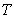- pulsar cycle time, days;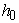- amplitude of a gravitational wave.

Our method of identification [3, 4, 5, 6] considerably differs and the purpose of article – to show a wavelet analysis technique, on the example of Table 1, and detection of steady and obviously nonlinear wave regularities of change of amplitude of GW depending on a cycle time of double pulsars. Thereby the hypothesis of the splashing Universe  will be proved.

Wavelet (splash) — is mathematical function. The English word "wavelet" is meant in translation by "a small wave". Wavelets — this family of functions, "the waves going one after another". Abscissa axis can be not only time (a pulsar cycle time), but also any other physical quantity. Wavelets has the accurate amplitude-frequency characteristic.

Thus harmonious wavelet any nature and an asymmetric form has a formula appearance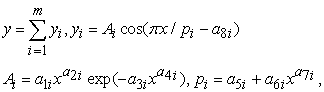(1)

where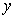- an indicator (amplitude of gravitational waves),- component number,- number of members of model (1),- an explaining variable,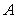- amplitude (half) of wavelet (axis),- a half-cycle of fluctuation (axis),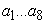- the parameters of model (1) identified on basic data from Table 1.

The signal - is a material data carrier. And information is understood by us as measure of interaction. The signal can be generated, but its reception isn't obligatory.

### 2. Identification Structural

Descartes assumed existence of the one and only algebraic equation suitable as version of the decision for any types of the integrated equations. Hilbert dreamed of invariants from which as from bricks this universal equation will gather. Our universal invariants are given in Table 2.

They are grouped in the principle "from simple to difficult". In fact, fragments and the biotechnical law are "Hilbert's bricks" for construction, during process of structural and parametrical identification, an additive design of required statistical model with trends and wavelets. Invariants of oscillatory indignations in the form of asymmetric wavelet-signals also include constructs from Table 2 as amplitude (half) and a half-cycle.

#### Table 2. Mathematical constructs in the form of stable laws to build a statistical model

In Table 2 the most meeting invariants (fragments) are shown. At them ahead can be located operational constants «+» or «-». Six stable distribution laws are special cases of biotech law, shown at the bottom of Table 2. In the title of the law the word «biotechnical» means that we adhere to the ideas of V.I. Vernadsky about the space of the functions of life. This is proved  by the fact that the Fibonacci series is a kind of «relative» of a number of positive primes. If you know the background of the formation of heuristic numeric field (table model), it is quite possible semantic decoding each wavelet signal whose wavelet (wave function) in its design contains certain mathematical invariants from Table 2.

All known laws of distribution are particular cases of the biotech law that is shown in detail in the tutorial .

Stable laws and the laws based on them make the selection equation for identification on statistical data (numeric fields) is quite meaningful, and therefore probabilistic modeling is only at random search software environment CurveExpert type values in the desired model. Therefore, identification of the theory first stage (selection of random structure of the equation) is excluded and only the second stage - the random identification of the model parameters.

Identification of the structure of the model is carried out by treating the initial data as follows:

- first identify deterministic nonlinear patterns;

- then add to these trend patterns vibration disturbances.

### 3. Parametric Identification

It was performed in a software environment CurveExpert-1.40 and information identification technology used by students (future bachelors and masters), as well as graduate and doctoral students.

On the methodology through examples structural-parametric identification is shown in detail in the book .

Selecting the desired model of the structure, which is an algebraic solution for the unknown Descartes primitive by wave equations having variable amplitude and half-life (half the frequency as the inverse of the half-period) oscillatory perturbation of the research object is made of stable laws (invariants), shown in Table 2.

Process of parametrical identification automatically stops on a condition of achievement of parameters of model of some minimum increment and stops the user at achievement by designed model of an error of measurements.

### 4. Levels of Adequacy of Revealed Regularities

Table 3 shows the ranges of the correlation coefficient as a measure of the adequacy of the model.

#### Table 3. Levels of crowding factor relations

The existing range of quantification closeness of the connection between the factors taken (no communication, weak and strong coupling) is very rude.

Criterion (a quantitative measure) identification becomes a measurement error in the preparation of a number field, i.e. table source data for other phases of identification. For example, prime numbers and their series  no have error measurement error: they are absolutely reliable, high-quality and reliable. Other types of basic data have different original error. Thus, numerical mathematical objects - to identify the best law-invariants.

In second place are precision measurements in astronomy, physics, engineering and technology and other fields of science. In third place stand biological objects (we - the trees), and their behavior has clearly manifested an oscillatory character, so linear and linear zed models biologists do not fit. The highest error are socio-economic dimensions because of their high subjectivity.

Therefore, we have proposed for technical experiments, in which the measurement error does not exceed 5%, the other scale (the third column of Table 3).

However, it became clear that the scale levels of adequacy is also insufficient.

For many natural (biological) objects and results precision physical measurements had to introduce two interval adequacy level of the fourth column of Table 2, that the world was made only in the simulation distributions series primes . And for genetic engineering, according to article1, we had to introduce another level of adequacy, which we opened only for whole series of whole simple numbers.

### 5. The Concept of Modeling by Statistical Sampling

Statistical sampling - a multifactor numeric field, formalized as a table model. This definition is substantially supplemented compared with the tables of statistical surveys. Not necessarily all of the cells of the table should be filled with numbers.

The table model has optional heuristic explanations. As a rule, the authors of measurement resulting in their publications the data tables, give incorrect meaningful interpretation.

This phenomenon formalization due to the fact what table of the results of measurements, even if it is made by the authors correctly, can not be meaningfully interpreted without holding a factor analysis  with mathematical modeling links between the pairs of factors to identify binary relations.

Then primary there is a tabular model (an initial numerical field) which is estimated on an error of the carried-out measurements, and secondary is sought complicated algebraic equation (in the sense of Descartes), composed of invariants of Table 2 (that is Hilbert's bricks).

This process – statistical identification. Primitive in the form of the unknown integrated equation becomes not necessary though, maybe, someone and will manage to receive integrals on our models.

This would be a great creation, as Maxwell's equations for electromagnetism.

### 6. The Determined Model

Generally not the wave model (a trend, a tendency) contains the sum of two biotechnical laws [3-6] and receives a type of the equation(2)

where- a trend (tendency),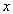- an explaining variable,- parameters of model (2).

Each parameter of model (2) makes physical sense which was in detail explained in our many publications. By the principle "from simple to difficult", in relation to 10 pulsars, in Table 4 private forms of the general statistical regularity (2) are given.

The first option is average value (differs from an arithmetic average on non-Gaussian distributions) and shows lack of factorial communication. That it not the average, is visible from the second option on linear model. However further complication of linear model in the form of a polynom doesn't make physical sense as even economists approximately since about 1995 ceased to publish articles with the square equation.

#### Table 4. Parameters of a trend (2) influences of a cycle time of pulsars on amplitude of gravitational waves

The law Laplace-Mandelbrot (option 3) makes physical sense and therefore significantly differs from a hyperbole of the indicative law. But it is rough for identification and therefore we had to enter in a formulathe third parameter - intensity of death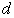. But it on an example from Table 1 isn't identified that points to a polynomials of required model. Strong adequacy with coefficient of correlation 0,7216 was received by a formula (option 5) with 's two laws, and the second member received a negative sign and therefore gives negative second essence of formation of gravitational waves.

At identification always it is necessary to try to bring a design of required regularity to completeness, i.e. there has to be a formula with two modifications (to a full design) Laplace's law.

For using the reader our technique we will write down a trend with all significant figures received in listing (Figure 1 and Table 5) from the program CurveExpert-1.40 environment in the form of a formula(3)Figure 1. Model of trend change of amplitude of a gravitational wave from the period at 10 pulsars

The graph visible «energy pit», from which the GW amplitude gradually fetched, but then again slowly decreases. If you want to get high-adequate model, then you have to calculate the relative error (Table 5) in each point of measurement.

The remains are calculated on expression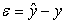, where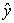- the actual value of the indicator according to the Table 1. Then the relative error will be equal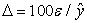.

#### Table 5. Sources of gravitational waves (deterministic model)

The maximum relative erroris equal 95,66% and such adequacy of required statistical model even if in one point of measurements, it is obviously insufficient.

### 7. Wave Functions

On the remains from not wave model on a formula (3) the wavelet signal with variables amplitude and the period (frequency) of fluctuation was received (Figure 2).

Any oscillatory indignation in a universe happens, apparently, for any adaptation of natural objects among themselves. From the schedule in Figure 2 it is accurately visible that oscillatory indignation of system of pulsars happens in the range of a cycle time of pulsars 0.1-1.0 days.

From Table 1 we notice that in this interval there are seven star systems, except three pulsars PSR J0700+6418, PSR J1829+2456 and PSR J1439−5501. They are located to the right of the pointer point (this point on conditional border between definiteness and uncertainty), equal 1.0

But then it turns out that the earth day have explicit cosmological meaning.

After merging with the trend model (3) the resulting graph (Figure 3) according to the formula(4)Here:- amplitude (half) of oscillatory indignation of an indicator (GW amplitude);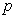- half-cycle of oscillatory adaptation of pulsars.

In this expressionis the frequency of oscillation, but to bind to the dimension of the abscissa is more convenient half-period.

Thus, at the first stage of change of amplitude of GW in the range from zero till 0.10225 days there is a downfall. But collapse doesn't occur as at the second stage pulsars get to the «shake-ups» mode for increase of amplitude of GW. However, after completion of oscillatory adaptation, at the third stage there is a slow decrease in amplitude of GW.

The error of identification of model (4) sharply decreased, and it is shown in Table 6.

#### Table 6. Sources of gravitational waves (trend + wavelet)

Gravitational oscillatory indignation gives the maximum relative error of model (4) only 6.44%.

Two pulsars marked in Table 6, were given the opportunity to identify the parameters of the second wavelet signal.

But in the beginning we will compare the equation (4) on change of amplitude of GW with well-known and in physics 's law. Under this law, the Laplace-Mandelbrot (version 5 of Table 3) should be collapse of the Universe, as shown in Figure 4, the formula of the form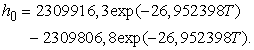(5)
Figure 4. Collapse with achievement of amplitude of a gravitational wave to zero in the range of a cycle time of pulsars from zero to 0.4 days under Laplace-Mandelbrot's two exponential laws

Equation (5) «works» only in the interval period pulsars 0.0-0.4 day. Within this model the activity death 26.952398 same two cones of causality (positive and negative causality equally responsive. As for the conditionturns out a small difference in amplitude strong aperiodic oscillation of GW just 2309916.3 - 2309806.8 = 106.0 units.

Then well-known in physics equation Mandelbrot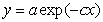is clearly inaccurate.

### 8. Model Splashing Universe

The remainder of the model (4) was obtained by the second asymmetric wavelet signal whose graph is shown in Figure 5.

The interval of coverage of a cycle time of pulsars in this second fluctuation changes from 0.4 to 2.2-2.3 days. Therefore two fluctuations are imposed at each other in the range of 0.4-1.0 days.

Together with a formula (4) the look model turned out (Figure 6)(6)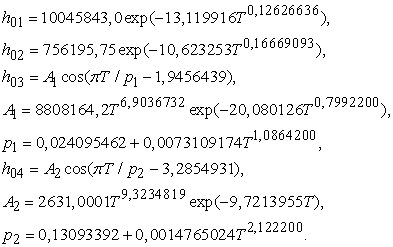From formula (6) we see that the amplitude of the oscillation periods GW providedto equal the first wavelet 2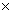0.024095462 = 0.048190924 and second asymmetric oscillatory waves 20.13093392 = 0.26186784units (it is necessary to take into account the multiplier 10-22).

Figure 6. The schedule of model (6) of change of amplitude of gravitational waves from a cycle time of 10 pulsars. The attitude of the second fluctuation towards the first wavelet will be equal 5.43398

Frequency of two gravitational waves, provided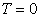the infinitely rapidly rotating theoretical pulsar equal 20.750805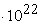and 3.818721.

### 9. Analysis of the Model Splashing Universe

In the beginning we will write down model (6) in a matrix form (that gives very compact record of formulas) as it is shown in Table 7 (RTG – the relativistic theory of gravitation). Thus we will write down numbers of components of the general model (1) not on a course of process of identification, and on groups in the form of two cones of causality from the relativistic theory of gravitation (RTG).

Further calculated in Excel each component of model (6), and then found individual share.

#### Table 7. Parameters of the wave equation (6) distributions of 10 pulsars on influence of the period of their circulation on amplitude of gravitational waves on the general model (1)

At preset values of fundamental constants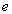and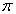with 18 signs after a comma by opportunities of the program environment CurveExpert-1.40 the formula (6) allows to calculate GW amplitude in an interval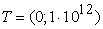. But, after a cycle time of 81760 days, it is almost possible to accept.

Under a conditionwe receive value of amplitude GW equal 9.2896472510-18.

From data of Table 4 we will take the average amplitude of GW of one pulsar, equal 1,82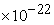. Then, on the approximate number of pulsars in the Universe if they are synchronous, it is possible to learn the general amplitude of GW from all star systems. From results of calculations the following two features were noticeable.

First, after a cycle time in 81760 days – 10 cycles of circulation core of the Sun round themselves – within an error of measurements from model (6) remain only the first component, and other three come nearer to zero. Then we can claim that «… in the Universe there have to be cold objects of big masses …»  with a cycle time at limits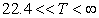years. Secondly, in the range of a cycle time of 0.1-1.0 days the maximum of individual share in amplitudeof GW separate a component in the form of oscillatory indignations is observed. Direct calculation of the expression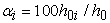obtained, that the maxima are at an optimum level(Table 8).

#### Table 8. Optimum cycle times at the maximum frequency rate of members (6)

Comparison to Table 1 shows that most close in maxima of two cones of causality of RTG there is a pulsar № 9 PSR J1906+0746 with a cycle time of 0.16599 days and amplitude of gravitational waves 0,71.

The difference from an optimum cycle time makes all 100 (0.17037 – 0.16599) / 0.17037 = 2.57%.

Therefore, further study of this pulsar appears to give the opportunity to discover the maximum multiplicity of cones of causality (I – 438.922) and (II – 437.922) and by that to prove dualism of emergence of a visible matter in our Universe.

In addition we can note the third feature.

Valueof days equally 0.1703724 = 4.09 hours. It is known that more than four billions years ago Earth rotated with such frequency. Then it turns out that in these times in the young Solar system the maximum operated two causality RTG.

### 10. Conclusion

The example shows that the simulation of the statistical precision reveals stable patterns.

The method of identification of wave functions in the form of wavelet signals of the asymmetric form, shown in this article on the example of a set from 10 pulsars, gives the chance of substantial judgment of the oscillatory indignations happening in natural processes. Thus determined components of the revealed statistical model appear the strong aperiodic fluctuations having a half-cycle of indignation much more bigger of an interval of measurements of the explaining variable.

Two causality cone is formed by four members of general statistical model. Each causality cone contains one trend in the world as a modified 's law and one wavelet.

At preset values of fundamental constantsandwith 18 signs after a comma by opportunities of the program environment CurveExpert-1.40 the formula (6) allows to calculate GW amplitude in an interval. But, after a cycle time of 81760 days, it is almost possible to accept.

Under a conditionwe receive value of amplitude GW equal 9.2896472510-18.

To prove a formula (6) as the mathematical law of behavior of pulsars, it is necessary to identify repeatedly it on new sets of sources of gravitational waves in the form of addition with again studied star systems.

Therefore we will wait from experts fuller, in comparison by Table 1 (both by number of star systems, and on the number of the studied indicators), the table with parameters of the studied pulsars.

### References

  Brian Cox. Wonders of the universe. : Eksmo, 2012. 256 pages.In article  S.F. Levin Statistical methods of the solution of measuring problems of cosmology and gravitation // International session conference of Section of nuclear physics of Office of physical sciences of the Russian Academy of Sciences "Physics of fundamental interactions": 12-16.11.2012. Moscow: National Research Nuclear University “MEPhI”, 2012. 75 pages.In article  P.M. Mazurkin, “Wavelet Analysis of a Number of Prime Numbers.” American Journal of Numerical Analysis, vol. 2, no. 2 (2014): 29-34.In article  P.M. Mazurkin. Patterns of primes. Germany: Palmarium Academic Publishing, 2012. 280 p.In article  P.M. Mazurkin, “Stable Laws and the Number of Ordinary.” Applied Mathematics and Physics, vol. 2, no. 2 (2014): 27-32.In article CrossRef  P.M. Mazurkin, A.S. Filonov, Mathematical modeling. Identification univariate statistical regularities: tutorial. Yoshkar-Ola, Mari State Technical University, 2006. 292 pages.In article